• Computation of the Generalized Mittag-Leffler Function and its Inverse in the Complex Plane
Sie sind hier: ICP » R. Hilfer » Publikationen

# Computation of the Generalized Mittag-Leffler Function and its Inverse in the Complex Plane

###### Abstract.

The generalized Mittag-Leffler function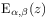has been studied for arbitrary complex argument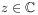and parameters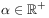and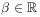. This function plays a fundamental role in the theory of fractional differential equations and numerous applications in physics. The Mittag-Leffler function interpolates smoothly between exponential and algebraic functional behaviour. A numerical algorithm for its evaluation has been developed. The algorithm is based on integral representations and exponential asymptotics. Results of extensive numerical calculations forin the complex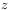-plane are reported here. We find that all complex zeros emerge from the point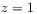for small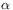. They diverge towards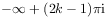for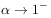and towards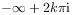for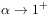(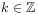). All complex zeros collapse pairwise onto the negative real axis for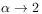. We introduce and study also the inverse generalized Mittag-Leffler function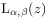defined as the solution of the equation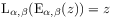. We determine its principal branch numerically.

###### Key words and phrases:
special functions of mathematical physics, fractional calculus, generalized Mittag-Leffler functions, numerical algorithms
PACS: 02.30.Gp, 02.60.Gf